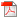# Gibbs Sampling Methods for Bayesian Quantile Regression

### 要約

This paper considers quantile regression models using an asymmetric Laplace distribution from a Bayesian point of view. We develop a simple and efficient Gibbs sampling algorithm for fitting the quantile regression model based on a location-scale mixture representation of the asymmetric Laplace distribution. It is shown that the resulting Gibbs sampler can be accomplished by sampling from either normal or generalized inverse Gaussian distribution. We also discuss some possible extensions of our approach, including the incorporation of a scale parameter, the use of double exponential prior, and a Bayesian analysis of Tobit quantile regression. The proposed methods are illustrated by both simulated and real data.

Key words: Asymmetric Laplace distribution, Bayesian quantile regression, double exponential prior, generalized inverse Gaussian distribution, Gibbs sampler, Tobit quantile regression(181.3KB)